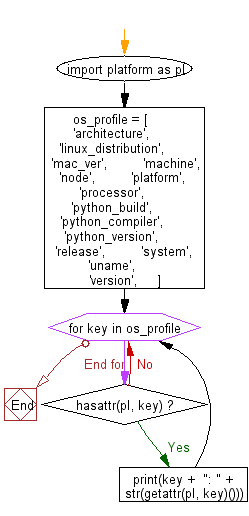﻿ Python: Display some information about the OS where the script is running - w3resource

# Python: Display some information about the OS where the script is running

## Python Basic - 1: Exercise-10 with Solution

Write a Python program to display some information about the OS where the script is running.

Sample Solution:

Python Code:

``````import platform as pl

os_profile = [
'architecture',
'linux_distribution',
'mac_ver',
'machine',
'node',
'platform',
'processor',
'python_build',
'python_compiler',
'python_version',
'release',
'system',
'uname',
'version',
]
for key in os_profile:
if hasattr(pl, key):
print(key +  ": " + str(getattr(pl, key)()))
``````

Sample Output:

```architecture: ('64bit', 'ELF')
linux_distribution: ('Ubuntu', '16.04', 'xenial')
mac_ver: ('', ('', '', ''), '')
machine: x86_64
node: 9a911676793b
platform: Linux-4.4.0-57-generic-x86_64-with-Ubuntu-16.04-xenial
processor: x86_64
python_build: ('default', 'Nov 17 2016 17:05:23')
python_compiler: GCC 5.4.0 20160609
python_version: 3.5.2
release: 4.4.0-57-generic
system: Linux
uname: uname_result(system='Linux', node='9a911676793b', release='4.4.0-57-generic', version='#78-Ubuntu SMP Fri Dec 9 23:50:32 UTC 2016', machine='x86_64', processor='x86_64')
version: #78-Ubuntu SMP Fri Dec 9 23:50:32 UTC 2016
```

Flowchart:Python Code Editor :

Have another way to solve this solution? Contribute your code (and comments) through Disqus.

What is the difficulty level of this exercise?

Test your Programming skills with w3resource's quiz.

﻿

## Python: Tips of the Day

Creates a list of elements, grouped based on the position in the original lists:

Example:

```def tips_zip(*args, fill_value=None):
max_length = max([len(lst) for lst in args])
result = []
for i in range(max_length):
result.append([
args[k][i] if i < len(args[k]) else fillvalue for k in range(len(args))
])
return result
print(tips_zip(['a', 'b'], [1, 2], [True, False]))
print(tips_zip(['a'], [1, 2], [True, False]))
print(tips_zip(['a'], [1, 2], [True, False], fill_value = '1'))
```

Output:

```[['a', 1, True], ['b', 2, False]]
[['a', 1, True], [None, 2, False]]
[['a', 1, True], ['1', 2, False]]
```